# Calogero-Moser-Krichever system

(diff) ← Older revision | Latest revision (diff) | Newer revision → (diff)

Calogero–Moser–Sutherland–Krichever system

A finite-dimensional Hamiltonian system which is algebraically completely integrable (cf. Completely-integrable differential equation). It admits several generalizations, which will be indicated below. During the enormous activity on mathematical aspects of integrable systems and soliton equations, starting in the late 1960{}s, some prototypes that represent the main features have emerged. For example, the Korteweg–de Vries equation and the non-linear Schrödinger equation for inverse scattering [a8], rank-perturbations for algebraic complete integrability [a19], [a20], the Kadomtsev–Petviashvili equation (cf. Soliton) for Grassmannians and Schur functions [a30], and the modified Korteweg–de Vries equation for representation theory [a6], [a15].

As a prototype, the Calogero–Moser–Krichever system possesses all the standard features of algebraic complete integrability: 1) a Lax pair, spectral curve, and Abelian integrals; 2) a connection with soliton equations; 3) an-matrix; 4) a geodesic-motion interpretation. However, it has also more unique features: 5) a link with elliptic functions; 6) a more sophisticated interpretation of the Lax pair, giving a link with the Hitchin system, which is a more powerful source of algebraic complete integrability over moduli spaces of vector bundles; 7) the bispectral property.

A brief illustration of these terms follows.

1) The system has a Hamiltonian function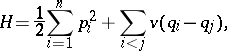whereare position/momentum variables (all quantities are complex numbers) andis an even function. Ifandare the-matrices with entries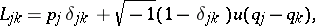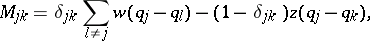whereis odd and,are even, then the equation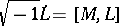implies,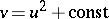, as well as a functional equation forand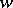with solutionsThese three cases are referred to as rational (Calogero–Moser system), trigonometric (Calogero–Sutherland system) and elliptic (Calogero–Moser–Krichever system), respectively; the first two can be viewed as limits of the third as one or both periods of the Weierstrass function(cf. Weierstrass elliptic functions) tend to infinity. In each case, the invariants,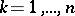, are (generically) functionally independent and in involution (cf. Integrals in involution), so that the system is completely integrable. This was the first example [a21] of a Lax pair with as parameter a function over a curve of genus(generalizations are still (1996) quite rare, cf. [a10] for hyperelliptic parameters).

2) By interpolating an eigenvector ofinto a Baker–Akhiezer function, it was shown in [a21] that the solutions correspond to elliptic (in) solutions of the Kadomtsev–Petviashvili equation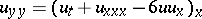. The first breakthrough in this respect was made in [a1], concerning Korteweg–de Vries solutions and Lamé equations (cf. also Lamé equation); moduli spaces of the corresponding algebro-geometric configurations (tangential covers) were described in [a31] and their density was detected in [a3].

3) Several models of the-matrix, both dynamic and non-dynamic, recently (1990{}s) became available (for a most complete set of references see [a11]); however a finite-dimensional geodesic motion interpretation 4) has only been achieved for the rational and trigonometric cases [a17].

5) S.P. Novikov posed the following question: Which algebro-geometric Kadomtsev–Petviashvili solutions can be expressed in terms of elliptic functions (cf. also Elliptic function)? These non-trivially overlap with elliptic solitons and a state-of-the-art report can be found in [a22].

6) is the deep theory that ensues if the moving data is viewed as a rank-bundle over the elliptic curve, cf. [a4], [a9].

Lastly, 7) is an extra property of the Baker function in the rational case, defined by F.A. Grünbaum [a7] in connection with computerized tomography (roughly stated, the Baker function is both an- and a-eigenfunction for a pair of operators with eigenvalues that are functions of,, respectively); its manifestation for the matrixabove (cf. [a1]) is investigated in [a16].

A final word about generalizations: [a27] adapts the geodesic problem 4) to other groups and metrics; [a28] defines a relativistic Calogero–Moser–Krichever system; [a24] and [a25] provide discretized versions of the Calogero–Moser–Krichever and Ruijsenaars system, respectively; an Euler Calogero–Moser–Krichever system is related to a multi-component Kadomtsev–Petviashvili equation in [a23]; and the most recent application of the Calogero–Moser–Krichever system is that conjecturally it provides moduli for solutions to the Seiberg–Witten equations, [a5].

How to Cite This Entry:
Calogero-Moser-Krichever system. Encyclopedia of Mathematics. URL: http://encyclopediaofmath.org/index.php?title=Calogero-Moser-Krichever_system&oldid=18918
This article was adapted from an original article by E. Previato (originator), which appeared in Encyclopedia of Mathematics - ISBN 1402006098. See original article